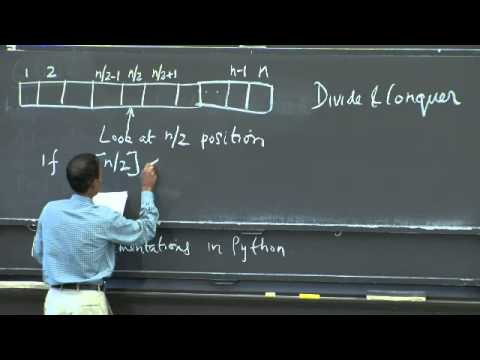MIT OpenCourseWare

# Introduction to Algorithms

This course provides an introduction to mathematical modeling of computational problems. It covers the common algorithms, algorithmic paradigms, and data structures used to solve these problems. The course emphasizes the relationship between algorithms and programming, and introduces basic performance measures and analysis techniques for these problems. (from ocw.mit.edu )

Instructor

Computer science
2013

#### Lecture 01 - Algorithmic Thinking, Peak Finding